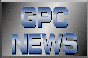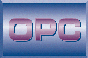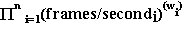Weighted Geometric Mean Selected
for Viewperf Composite Numbers

by Bill Licea-Kane

At its February 1995 meeting in Salt Lake City, a subcommittee within the OPC project group was given the task of recommending a method for deriving a single composite metric for each viewset running under the Viewperf benchmark. Composite numbers had been discussed by the OPC group for more than a year.

In May 1995, the OPC project group decided to adopt a weighted geometric mean as the single composite metric for each viewset.

### What is a Weighted Geometric Mean?Above is the formula for determining a weighted geometric mean, where "n" is the number of individual tests in a viewset, and "w" is the weight of each individual test, expressed as a number between 0.0 and 1.0. (A test with a weight of "10.0%" is a "w" of 0.10. Note the sum of the weights of the individual tests must equal 1.00.)

The weighted geometric mean of CDRS-03, for example, is expressed by the following formula:

wgm-cdrs-03 =
(test10.50)*(test20.20)* (test30.15)*(test40.08)* (test50.05)*(test60.02)* (test70.00)

The same formula for the weighted geometric mean as expressed in a Microsoft Excel expression:

= (a1^0.05)*(b1^0.20)*(c1^0.15)*(d1^0.08)*(e1^0.05)*(f1^0.02)*(g1^0.00)

### Why the Weighted Geometric Mean?

The OPC subcommittee that recommended a method for determining composite numbers started with the description for assigning weights that is provided to each creator of a viewset: "Assign a weight to each path based on the percentage of time in each path..."

Given this description, the weighted geometric mean of each viewset is the correct composite metric. This composite metric is a derived quantity that is exactly as if you ran the viewset tests for 100 seconds, where test 1 was run for 100 × weight1 seconds, test 2 for 100 × weight2 seconds, and so on.

The end result would be the number of frames rendered/total time which will equal frames/second. It also has the desirable property of "bigger is better"; that is, the higher the number, the better the performance.

### Why Not Weighted Harmonic Mean?

Since the results of Viewperf are expressed as "frames/second," the subcommittee was asked why we did not choose the weighted harmonic mean. The weighted harmonic mean would have been the correct composite if the description published for Viewperf read as follows: "Assign a weight to each path based on the percentage of operations in each path..."

Given this description, the weighted harmonic mean would be as if you ran the viewset tests for 100 frames, where 100 × weight1 frames were drawn with test1, the next 100 × weight2 frames were drawn by test2, and so on. The 100 frames divided by the total time would be the weighted harmonic mean.

Since the weights for the viewsets were selected on percentage of time, not percentage of operations, we chose the weighted geometric mean over the weighted harmonic mean.

### What About Weighted Arithmetic Mean?

The weighted arithmetic mean is correct for calculating grades at the end of a school term. It is not correct for the situation we face here.

Consider for a moment a trivial example, where there are two tests, equally weighted in a viewset:

```
Test 1      Test2    Weighted Arithmetic Mean  		        50%          50%
System A	1.0         100.0		50.5
System B	1.1         100.0		50.55
System C	1.0         110.0		55.5```

System B is 10-percent faster at Test1 than System A. System C is 10-percent faster at Test2 than System A. But look at the weighted arithmetic means. System B's weighted arithmetic mean is only .1-percent higher than System A's, while System C's weighted arithmetic mean is 10-percent higher. Even normalization doesn't help here.

### Why Not Normalized Weighted Geometric Mean?

Here the OPC project group departs company from the nearly universal practice in benchmarking of normalizing test results. SPECint92, PLBsurf93 and Xmark93, for example, are all normalized results based on a variety of "reference" systems.

Since our weights were percentage of time and since the results from Viewperf are expressed in frames/sec, we were not obligated to normalize. Normalization introduces many issues of its own, starting with something as simple as how to select a reference system.

We invite readers to select two different systems whose results are published in this newsletter and to use each one as the reference system. You will discover quickly that the normalized weighted geometric means change only in absolute magnitude. If the weighted geometric mean of System B is 10-percent higher than System A, for example, the normalized weighted geometric mean of System B will be 10-percent higher than System A, no matter what reference system you choose.

### Is There a Disadvantage to Weighted Geometric Mean?

As with any composite, the weighted geometric mean can act as a "filter" for results; this introduces the danger that important information might be lost and inappropriate conclusions could be drawn. So, proper use of these composites is important. Use the composite as an additional piece of information. But also take a look at each individual test result in a viewset.

Please don't rely exclusively on any synthetic benchmark such as Viewperf. In the end, isn't actual application performance on an actual computer system what you are really attempting to find?

Bill Licea-Kane is responsible for graphics performance measurement within Digital Equipment Corp.'s Computer Systems Performance Group. He serves on all three GPC subcommittees and is chair of the PLB group. He can be reached by phone at 603-881-2804 or by e-mail at wwlk@perfit.zko.dec.com.

[APC Project] [GPC Home] [MBC Project] [OPC Project] [PLB Project] [XPC Project]